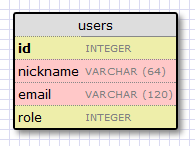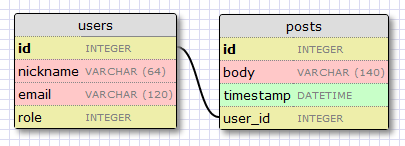12345 `import` `os ``basedir ``=` `os.path.abspath(os.path.dirname(__file__)) ``  ` `SQLALCHEMY_DATABASE_URI ``=` `'sqlite:///'` `+` `os.path.join(basedir, ``'app.db'``) ``SQLALCHEMY_MIGRATE_REPO ``=` `os.path.join(basedir, ``'db_repository'``)`

SQLALCHEMY_MIGRATE_REPO 是用来存储SQLAlchemy-migrate数据库文件的文件夹。

 12345678 `from` `flask ``import` `Flask ``from` `flask.ext.sqlalchemy ``import` `SQLAlchemy ``  ` `app ``=` `Flask(__name__) ``app.config.from_object(``'config'``) ``db ``=` `SQLAlchemy(app) ``  ` `from` `app ``import` `views, models`id字段通常作为主键的形式用在所有的models里面，每个在数据库中的user都有一个指定的唯一id值。幸运的是，这些都是自动的，我们只需要提供一个id字段。

nickname和email字段被定义为string类型，他们的长度也已经被指定，这样可以节省数据库存储空间。

 12345678910111213 `from` `app ``import` `db ``  ` `ROLE_USER ``=` `0``ROLE_ADMIN ``=` `1``  ` `class` `User(db.Model): ``  ``id` `=` `db.Column(db.Integer, primary_key ``=` `True``) ``  ``nickname ``=` `db.Column(db.String(``64``), index ``=` `True``, unique ``=` `True``) ``  ``email ``=` `db.Column(db.String(``120``), index ``=` `True``, unique ``=` `True``) ``  ``role ``=` `db.Column(db.SmallInteger, default ``=` `ROLE_USER) ``  ` `  ``def` `__repr__(``self``): ``    ``return` `''` `%` `(``self``.nickname)`

User类把我们刚刚创建的几个字段定义为类变量。字段使用db.Column类创建实例，字段的类型作为参数，另外还提供一些其他可选参数。例如，标识字段唯一性和索引的参数.

__repr__方法告诉Python如何打印class对象，方便我们调试使用。

 123456789101112 `#!flask/bin/python ``from` `migrate.versioning ``import` `api ``from` `config ``import` `SQLALCHEMY_DATABASE_URI ``from` `config ``import` `SQLALCHEMY_MIGRATE_REPO ``from` `app ``import` `db ``import` `os.path ``db.create_all() ``if` `not` `os.path.exists(SQLALCHEMY_MIGRATE_REPO): ``  ``api.create(SQLALCHEMY_MIGRATE_REPO, ``'database repository'``) ``  ``api.version_control(SQLALCHEMY_DATABASE_URI, SQLALCHEMY_MIGRATE_REPO) ``else``: ``  ``api.version_control(SQLALCHEMY_DATABASE_URI, SQLALCHEMY_MIGRATE_REPO, api.version(SQLALCHEMY_MIGRATE_REPO))`

 1 `.``/db_create``.py`

 123456789101112131415 `#!flask/bin/python ``import` `imp ``from` `migrate.versioning ``import` `api ``from` `app ``import` `db ``from` `config ``import` `SQLALCHEMY_DATABASE_URI ``from` `config ``import` `SQLALCHEMY_MIGRATE_REPO ``migration ``=` `SQLALCHEMY_MIGRATE_REPO ``+` `'/versions/%03d_migration.py'` `%` `(api.db_version(SQLALCHEMY_DATABASE_URI, SQLALCHEMY_MIGRATE_REPO) ``+` `1``) ``tmp_module ``=` `imp.new_module(``'old_model'``) ``old_model ``=` `api.create_model(SQLALCHEMY_DATABASE_URI, SQLALCHEMY_MIGRATE_REPO) ``exec` `old_model ``in` `tmp_module.__dict__ ``script ``=` `api.make_update_script_for_model(SQLALCHEMY_DATABASE_URI, SQLALCHEMY_MIGRATE_REPO, tmp_module.meta, db.metadata) ``open``(migration, ``"wt"``).write(script) ``a ``=` `api.upgrade(SQLALCHEMY_DATABASE_URI, SQLALCHEMY_MIGRATE_REPO) ``print` `'New migration saved as '` `+` `migration ``print` `'Current database version: '` `+` `str``(api.db_version(SQLALCHEMY_DATABASE_URI, SQLALCHEMY_MIGRATE_REPO))`

 1 `.``/db_migrate``.py`

 1 `New migration saved as db_repository``/versions/001_migration``.py Current database version: 1`

 123456 `#!flask/bin/python ``from` `migrate.versioning ``import` `api ``from` `config ``import` `SQLALCHEMY_DATABASE_URI ``from` `config ``import` `SQLALCHEMY_MIGRATE_REPO ``api.upgrade(SQLALCHEMY_DATABASE_URI, SQLALCHEMY_MIGRATE_REPO) ``print` `'Current database version: '` `+` `str``(api.db_version(SQLALCHEMY_DATABASE_URI, SQLALCHEMY_MIGRATE_REPO))`

 1234567 `#!flask/bin/python ``from` `migrate.versioning ``import` `api ``from` `config ``import` `SQLALCHEMY_DATABASE_URI ``from` `config ``import` `SQLALCHEMY_MIGRATE_REPO ``v ``=` `api.db_version(SQLALCHEMY_DATABASE_URI, SQLALCHEMY_MIGRATE_REPO) ``api.downgrade(SQLALCHEMY_DATABASE_URI, SQLALCHEMY_MIGRATE_REPO, v ``-` `1``) ``print` `'Current database version: '` `+` `str``(api.db_version(SQLALCHEMY_DATABASE_URI, SQLALCHEMY_MIGRATE_REPO))`posts表包含一个必须的id,微博的内容body，还有一个时间戳。没有什么新东西，但是user_id字段值得解释下。

 1234567891011121314151617181920212223 `from` `app ``import` `db ``  ` `ROLE_USER ``=` `0``ROLE_ADMIN ``=` `1``  ` `class` `User(db.Model): ``  ``id` `=` `db.Column(db.Integer, primary_key ``=` `True``) ``  ``nickname ``=` `db.Column(db.String(``64``), unique ``=` `True``) ``  ``email ``=` `db.Column(db.String(``120``), unique ``=` `True``) ``  ``role ``=` `db.Column(db.SmallInteger, default ``=` `ROLE_USER) ``  ``posts ``=` `db.relationship(``'Post'``, backref ``=` `'author'``, lazy ``=` `'dynamic'``) ``  ` `  ``def` `__repr__(``self``): ``    ``return` `''` `%` `(``self``.nickname) ``  ` `class` `Post(db.Model): ``  ``id` `=` `db.Column(db.Integer, primary_key ``=` `True``) ``  ``body ``=` `db.Column(db.String(``140``)) ``  ``timestamp ``=` `db.Column(db.DateTime) ``  ``user_id ``=` `db.Column(db.Integer, db.ForeignKey(``'user.id'``)) ``  ` `  ``def` `__repr__(``self``): ``    ``return` `''` `%` `(``self``.body)`

 1 `.``/db_migrate``.py`

 1 `New migration saved as db_repository``/versions/002_migration``.py Current database version: 2`

Windows下:

 1 `>>> ``from` `app ``import` `db, models >>>`

 1234 `>>> u ``=` `models.User(nickname``=``'john'``, email``=``'john@email.com'``, role``=``models.ROLE_USER) ``>>> db.session.add(u) ``>>> db.session.commit() ``>>>`

 1234 `>>> u ``=` `models.User(nickname``=``'susan'``, email``=``'susan@email.com'``, role``=``models.ROLE_USER) ``>>> db.session.add(u) ``>>> db.session.commit() ``>>>`

 123456789 `>>> users ``=` `models.User.query.``all``() ``>>> ``print` `users ``[, ] ``>>> ``for` `u ``in` `users: ``...   ``print` `u.``id``,u.nickname ``... ``1` `john ``2` `susan ``>>>`

 1234 `>>> u ``=` `models.User.query.get(``1``) ``>>> ``print` `u `` ``>>>`

 12345 `>>> ``import` `datetime ``>>> u ``=` `models.User.query.get(``1``) ``>>> p ``=` `models.Post(body``=``'my first post!'``, timestamp``=``datetime.datetime.utcnow(), author``=``u) ``>>> db.session.add(p) ``>>> db.session.commit()`

 12345678910111213141516171819202122232425 `# get all posts from a user ``>>> u ``=` `models.User.query.get(``1``) ``>>> ``print` `u `` ``>>> posts ``=` `u.posts.``all``() ``>>> ``print` `posts ``[] ``  ` `# obtain author of each post ``>>> ``for` `p ``in` `posts: ``...   ``print` `p.``id``,p.author.nickname,p.body ``... ``1` `john my first post! ``  ` `# a user that has no posts ``>>> u ``=` `models.User.query.get(``2``) ``>>> ``print` `u `` ``>>> ``print` `u.posts.``all``() ``[] ``  ` `# get all users in reverse alphabetical order ``>>> ``print` `models.User.query.order_by(``'nickname desc'``).``all``() ``[, ] ``>>>`

 12345678910 `>>> users ``=` `models.User.query.``all``() ``>>> ``for` `u ``in` `users: ``...   db.session.delete(u) ``... ``>>> posts ``=` `models.Post.query.``all``() ``>>> ``for` `p ``in` `posts: ``...   db.session.delete(p) ``... ``>>> db.session.commit() ``>>>`

### 01 Get提交

（1）前端：

``````<script src="{{url_for('static',filename='js/jquery.js')}}"></script>
<!--Get请求-->
<script>
var data={
'name':'kikay',
'age':18
}
\$.ajax({
type:'GET',
url:'{{url_for("test.test_get")}}',
data:data,
dataType:'json',//希望服务器返回json格式的数据
success:function(data){
}
});
</script>``````
• 1
• 2
• 3
• 4
• 5
• 6
• 7
• 8
• 9
• 10
• 11
• 12
• 13
• 14
• 15
• 16
• 17
• 18

（2）后端：

``````@test.route('/test_get/',methods=['POST','GET'])
def test_get():
#获取Get数据
name=request.args.get('name')
age=int(request.args.get('age'))
#返回
if name=='kikay' and age==18:
return jsonify({'result':'ok'})
else:
return jsonify({'result':'error'})``````
• 1
• 2
• 3
• 4
• 5
• 6
• 7
• 8
• 9
• 10

### 02 Post方式

（1）前端：

``````<script src="{{url_for('static',filename='js/jquery.js')}}"></script>
<!--Post请求-->
<script>
var data={
'name':'kikay',
'age':18
}
\$.ajax({
type:'POST',
url:'{{url_for("test.test_post")}}',
data:data,
dataType:'json',//希望服务器返回json格式的数据
success:function(data){
}
});
</script>``````
• 1
• 2
• 3
• 4
• 5
• 6
• 7
• 8
• 9
• 10
• 11
• 12
• 13
• 14
• 15
• 16
• 17

（2）后端：

``````@test.route('/test_post/',methods=['POST','GET'])
def test_post():
#获取POST数据
name=request.form.get('name')
age=int(request.form.get('age'))
#返回
if name=='kikay' and age==18:
return jsonify({'result':'ok'})
else:
return jsonify({'result':'error'})``````
• 1
• 2
• 3
• 4
• 5
• 6
• 7
• 8
• 9
• 10

### 03 Json提交

（1）前端：

``````

发表于 2019-10-09 10:48

阅读 ( 60 )
``` ```
``` ```
``` <<上一篇 下一篇>> 0 条评论 请先 登录 后评论 ```
```幸福清风_csdn 0 篇文章 作家榜 »AI君 10 文章Tzung-Wen Liau 0 文章blairan 0 文章rookie 0 文章陈凯 0 文章huanxue 0 文章admin 0 文章Lzs1998_csdn 0 文章 ```
``` ```
``` ```
``` AI教程网| 联系我们| 浙ICP备14002151号-2 Copyright ©2018-2023 ainoob.cn 发送私信 发给: 内容: var is_login = Boolean("");如果觉得我的文章对您有用，请随意打赏。你的支持将鼓励我继续创作！\$(document).ready(function() { var article_id = "10071"; /*评论默认展开*/ load_comments('article',article_id); \$("#comments-article-"+article_id).collapse('show'); /*评论提交*/ \$(".comment-btn").click(function(){ var source_id = \$(this).data('source_id'); var source_type = \$(this).data('source_type'); var to_user_id = \$(this).data('to_user_id'); var token = \$(this).data('token'); var content = \$("#comment-"+source_type+"-content-"+source_id).val(); add_comment(token,source_type,source_id,content,to_user_id); \$("#comment-content-"+source_id+"").val(''); }); /*文章推荐*/ \$("#support-button").click(function() { var btn_support = \$(this); var source_type = btn_support.data('source_type'); var source_id = btn_support.data('source_id'); var support_num = parseInt(btn_support.data('support_num')); \$.get('/support/' + source_type + '/' + source_id, function (msg) { if (msg == 'success') { support_num++ } btn_support.html(support_num+' 已推荐'); btn_support.data('support_num', support_num); }); }); /*收藏问题或文章*/ \$("#collect-button").click(function(){ \$("#collect-button").button('loading'); var source_type = \$(this).data('source_type'); var source_id = \$(this).data('source_id'); \$.get('/collect/'+source_type+'/'+source_id,function(msg){ \$("#collect-button").removeClass('disabled'); \$("#collect-button").removeAttr('disabled'); if(msg=='collected'){ \$("#collect-button").html('已收藏'); }else{ \$("#collect-button").html('收藏'); } }); }); }); (function(){ var bp = document.createElement('script'); var curProtocol = window.location.protocol.split(':'); if (curProtocol === 'https') { bp.src = 'https://zz.bdstatic.com/linksubmit/push.js'; } else { bp.src = 'http://push.zhanzhang.baidu.com/push.js'; } var s = document.getElementsByTagName("script"); s.parentNode.insertBefore(bp, s); })(); ```